Test: Turing Machine: RE, REC & Undecidability- 2

# Test: Turing Machine: RE, REC & Undecidability- 2 - Computer Science Engineering (CSE)

Test Description

## 15 Questions MCQ Test Theory of Computation - Test: Turing Machine: RE, REC & Undecidability- 2

Test: Turing Machine: RE, REC & Undecidability- 2 for Computer Science Engineering (CSE) 2023 is part of Theory of Computation preparation. The Test: Turing Machine: RE, REC & Undecidability- 2 questions and answers have been prepared according to the Computer Science Engineering (CSE) exam syllabus.The Test: Turing Machine: RE, REC & Undecidability- 2 MCQs are made for Computer Science Engineering (CSE) 2023 Exam. Find important definitions, questions, notes, meanings, examples, exercises, MCQs and online tests for Test: Turing Machine: RE, REC & Undecidability- 2 below.
Solutions of Test: Turing Machine: RE, REC & Undecidability- 2 questions in English are available as part of our Theory of Computation for Computer Science Engineering (CSE) & Test: Turing Machine: RE, REC & Undecidability- 2 solutions in Hindi for Theory of Computation course. Download more important topics, notes, lectures and mock test series for Computer Science Engineering (CSE) Exam by signing up for free. Attempt Test: Turing Machine: RE, REC & Undecidability- 2 | 15 questions in 45 minutes | Mock test for Computer Science Engineering (CSE) preparation | Free important questions MCQ to study Theory of Computation for Computer Science Engineering (CSE) Exam | Download free PDF with solutions
 1 Crore+ students have signed up on EduRev. Have you?
Test: Turing Machine: RE, REC & Undecidability- 2 - Question 1

### Let M be a Turing Machine has Q = (q0, q1, q2, q3, q4} a set of states, input alphabets {0, 1}. The tape alphabets are {0,1, B, X, V). The symbol B is used to represent end of input string. The final state is q4. The transistions are as follows: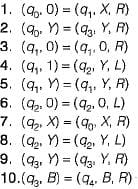Which of the following is true about M?

Detailed Solution for Test: Turing Machine: RE, REC & Undecidability- 2 - Question 1

Drawing the equivalent turing machine we have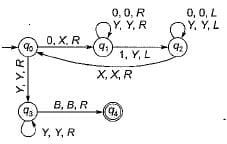This is a standard turing machine program for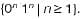Test: Turing Machine: RE, REC & Undecidability- 2 - Question 2

### If L1 and L2 are a pair of complementary languages. Which of the following statement is not possible?

Detailed Solution for Test: Turing Machine: RE, REC & Undecidability- 2 - Question 2

The correct statement follows from following theorem:
Theorem: If L1 and L2 is a pair of complementary language then either
• Both L1 and L2 are recursive.
• Neither L1, and L2 are recursively enumerable.
• One is recursively enumerable but not recursive, the other is not RE.
Option (b) is not possible, since if L1 is recursive, then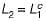will also be recursive.

Test: Turing Machine: RE, REC & Undecidability- 2 - Question 3

### Consider the following statements about L: (i) L is accepted by multi-tape turing machine M1. (ii) L is also accpted by a single tape turing machine M2. Which of the following statement is correct?

Detailed Solution for Test: Turing Machine: RE, REC & Undecidability- 2 - Question 3

While simulating multi-tape TM on a single tape TM, the head has to move at least 2k cells per move, where k is the number of tracks on single tape TM. Thus for k moves, we get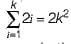which means quadratic slow down. Thus, acceptance by single-tape is slower by 0(n2).

Test: Turing Machine: RE, REC & Undecidability- 2 - Question 4

A TM M-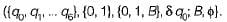The transition are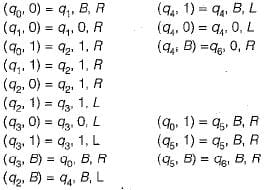What is the laugnage accepted by TM?

Detailed Solution for Test: Turing Machine: RE, REC & Undecidability- 2 - Question 4

The analysis of transition of TM, M shows that is accepts language 0*10*.

Test: Turing Machine: RE, REC & Undecidability- 2 - Question 5

Referring to Turing Machine in previous question, what will be the output when the input is
I1 = 0100 and I2 = 0010?

Detailed Solution for Test: Turing Machine: RE, REC & Undecidability- 2 - Question 5

The Turing Machine carries out the functions of ( m - n) in (0m 10n).
• When m≥n, the output is difference between m and n.
• when m < n, the output is blank.

Test: Turing Machine: RE, REC & Undecidability- 2 - Question 6

Nobody knows yets if p = NP consider the language L defined as follows: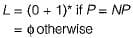Which of the following statement is true?

Detailed Solution for Test: Turing Machine: RE, REC & Undecidability- 2 - Question 6

L = (0 + 1 )* or {}. A trivial TM which accepts all words or another which rejects all. One of these two TMs will surely accept this language. So L is recursive.

Test: Turing Machine: RE, REC & Undecidability- 2 - Question 7

If the strings of a language L can be effectively enumerated in lexicographic (i.e. alphabetic) order which of the following statements is true?

Detailed Solution for Test: Turing Machine: RE, REC & Undecidability- 2 - Question 7

L is recursive iff L is enumerable in Lexicographic order (alphabetic).
It is also known that context free language is a subset of recursive languages.

Test: Turing Machine: RE, REC & Undecidability- 2 - Question 8

he diagnoalization language, Ld is a

Detailed Solution for Test: Turing Machine: RE, REC & Undecidability- 2 - Question 8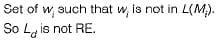Test: Turing Machine: RE, REC & Undecidability- 2 - Question 9

Time taken by one tape TM to simulate n moves of k-tape TM is

Detailed Solution for Test: Turing Machine: RE, REC & Undecidability- 2 - Question 9

Single tape turing machine can simulate n-moves of k-tape TM in O(n2).

Test: Turing Machine: RE, REC & Undecidability- 2 - Question 10

Consider the transition table of a TM given below. Here "b" represents the blank symbol.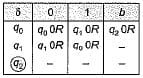Q. Which of the following strings will not accepted?

Detailed Solution for Test: Turing Machine: RE, REC & Undecidability- 2 - Question 10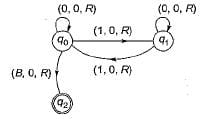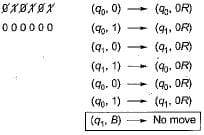Hence, not accepted.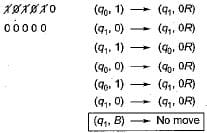Hence, also not accepted.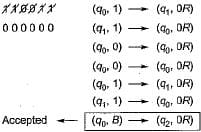Test: Turing Machine: RE, REC & Undecidability- 2 - Question 11

The given turing machine accepts

Detailed Solution for Test: Turing Machine: RE, REC & Undecidability- 2 - Question 11

It can be observed from the image that this machine will accept only those strings which contains even number of 1’s. And strings with odd number of 1 ’s are rejected.

Test: Turing Machine: RE, REC & Undecidability- 2 - Question 12

Ram and Shyam have been asked to show that a cartain problem II is NP-complete. Ram shows a polynomial time reduction from the 3-SAT problem to II and Shyam shows a polynomial time reduction from II to 3-SAT. Which of the following can be inferred from these reductions?

Detailed Solution for Test: Turing Machine: RE, REC & Undecidability- 2 - Question 12

Since 3-SAT problem is NP-complete and 3-SAT is polynomial time reducible to π hence π is is also NP-complete.

Test: Turing Machine: RE, REC & Undecidability- 2 - Question 13

Which of the following is true?

Detailed Solution for Test: Turing Machine: RE, REC & Undecidability- 2 - Question 13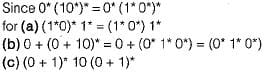Test: Turing Machine: RE, REC & Undecidability- 2 - Question 14

Both P and NP are closed under the operation of

Detailed Solution for Test: Turing Machine: RE, REC & Undecidability- 2 - Question 14

P and NP language are closed under union, intersection, kleene closure and concatenation, P is close under complement also while closure of NP under complement is still unsolved problem.

Test: Turing Machine: RE, REC & Undecidability- 2 - Question 15

L ∈ NP does not obviously imply that

Detailed Solution for Test: Turing Machine: RE, REC & Undecidability- 2 - Question 15

L ∈ NP does not imply that L' ∈ NP. Since closure of NP under complementation is as yet unresolved problem. Also L ∈ NP does not imply L' ∈ P, unless L ∈ P, which may or may not be so.

## Theory of Computation

18 videos|56 docs|44 tests
Information about Test: Turing Machine: RE, REC & Undecidability- 2 Page
In this test you can find the Exam questions for Test: Turing Machine: RE, REC & Undecidability- 2 solved & explained in the simplest way possible. Besides giving Questions and answers for Test: Turing Machine: RE, REC & Undecidability- 2 , EduRev gives you an ample number of Online tests for practice

## Theory of Computation

18 videos|56 docs|44 tests